There are a lot of algorithms that can prove mathematical identitie...
There are many representations of $e$ in this link: [List of repres...
Gradient descent (a.k.a steepest descent) is one of the best-known ...
Euler's equation is often called the most beautiful equation in mat...
To see the hydrogen spectral lines Rydberg was observing, see this ...
It can be shown that the digits of an irrational number do not term...
Super-exponential means that it grows even faster than an exponenti...
Since these are new results (conjectures) if you are able to prove ...
Fermat’s last theorem (a.k.a Fermat’s conjecture) states that no th...
Another cool example of such a collaborative project is SETI, searc...
We believe that sometimes the road can be just as important and ill...
This is probably one of the most important comments in this work an...
The Riemann hypothesis (a.k.a Riemann conjecture) is probably consi...
This figure shows one of the most powerful outcomes of the result. ...
Apery’s constant is the value of the Riemann zeta function at zeta=...
The idea here is that just like mathematicians study a lot of formu...
There are so many important constants in mathematics and other fiel...The Ramanujan Machine: Automatically Generated
Conjectures on Fundamental Constants
Gal Raayoni
1
, George Pisha
1
, Yahel Manor
1
, Uri Mendlovic
2
, Doron Haviv
1
, Yaron
1
, and Ido Kaminer
1
1
Technion - Israel Institute of Technology, Haifa 3200003, Israel
2
Google Inc., Tel Aviv 6789141, Israel
Abstract
Fundamental mathematical constants like
e
and
π
are ubiquitous in diverse ﬁelds of science, from
abstract mathematics and geometry to physics, biology and chemistry. Nevertheless, for centuries
new mathematical formulas relating fundamental constants have been scarce and usually discovered
sporadically. In this paper we propose a novel and systematic approach that leverages algorithms
for deriving new mathematical formulas for fundamental constants and help reveal their underlying
structure. Our algorithms ﬁnd dozens of well-known as well as previously unknown continued fraction
representations of
π
,
e
, and the Riemann zeta function values. Two new conjectures produced by our
algorithm, along with many others, are:
e = 3 +
1
4 +
2
5+
3
6+
4
7+...
,
4
π 2
= 3 +
1 · 3
5 +
2·4
7+
3·5
9+
4·6
11+...
We present two algorithms that proved useful in ﬁnding new results: a variant of the Meet-In-
The-Middle (MITM) algorithm and a Gradient Descent (GD) tailored to the recurrent structure
of continued fractions. Both algorithms are based on matching numerical values and thus ﬁnd
new conjecture formulas without providing proofs and without requiring prior knowledge on any
mathematical structure. This approach is especially attractive for fundamental constants for which no
mathematical structure is known, as it reverses the conventional approach of sequential logic in formal
proofs. Instead, our work presents a new conceptual approach for research: computer algorithms
utilizing numerical data to unveil new internal structures and conjectures, thus playing the role of
mathematical intuition of great mathematicians of the past, providing leads to new mathematical
research.
Code available at: http://www.ramanujanmachine.com/ or https://github.com/AnonGit90210/RamanujanMachine.
11 Introduction
Fundamental mathematical constants such as
e
,
π
, the golden ratio
ϕ
, and many others play an instrumental
part in diverse ﬁelds such as geometry, number theory, calculus, fundamental physics, biology, and ecology
[
1
]. Throughout history simple formulas of fundamental constants symbolized simplicity, aesthetics, and
mathematical beauty. A couple of well-known examples include Euler’s identity
e
+ 1 = 0 or the
continued fraction representation of the Golden ratio:
ϕ =
1
1 +
1
1+
1
1+...
. (1)
The discovery of such Regular Formulas (RFs)
1
was often sporadic and considered an act of math-
ematical ingenuity or profound intuition. One prominent example is Gauss’ ability to see meaningful
patterns in numerical data that led to new ﬁelds of analysis such as elliptic and modular functions and
to the hypothesis of the Prime Number Theorem. He is even famous for saying “I have the result, but I
do not yet know how to get it” [
2
], which emphasizes the role of identifying patterns and RFs in data as
enabling acts of mathematical discovery.
In a diﬀerent ﬁeld but in a similar manner, Johannes Rydberg’s discovery of his formula of hydrogen
spectral lines [
3
], resulted from his data analysis of the spectral emission by chemical elements:
λ
1
=
R
H
(
n
2
1
n
2
2
), where
λ
is the emission wavelength,
R
H
is the Rydberg constant,
n
1
and
n
2
are the
upper and lower quantum energy levels respectively. This insight, emerging directly from identifying
patterns in the data, had profound implications on modern physics and quantum mechanics.
Unlike measurements in physics and all other sciences,
mathematical
constants can be calculated
to an arbitrary precision (number of digits) with an appropriate formula, thus providing an
absolute
ground truth
. In this sense, mathematical constants contain an unlimited amount of data (e.g. the
inﬁnite sequence of digits in an irrational number), which we propose to use as a ground truth for ﬁnding
new RFs. Since the fundamental constants are universal and ubiquitous in their applications, ﬁnding such
patterns can reveal new mathematical structures with broad implications, e.g. the Rogers-Ramanujan
continued fraction (which has implications on modular forms) and the Dedekind η and j functions [4, 5].
Consequently, having a
systematic
method to derive new RFs can help research in many ﬁelds of science.
In this paper, we establish a novel method to learn mathematical relations between constants and we
present a list of new conjectures found using this method. While the method can be leveraged for many
forms of RFs, we demonstrate its potential with equations of the form of generalized continued fractions
(GCFs):
x = a
0
+
b
1
a
1
+
b
2
a
2
+
b
3
a
3
+...
, (2)
where
a
n
, b
n
Z
for
n
= 1
,
2
, . . .
are partial numerators and denominators respectively. GCFs in which
the partial numerators and denominators follow a closed-form expression like a polynomial have been of
interest to mathematicians for centuries and still are today, e.g. William Broucker’s π representation 
or [1, 7, 8].
We demonstrate our approach by ﬁnding identities between a GCF and the value of a rational function
at a fundamental constant. For simplicity, enumeration and expression aesthetics, we limit ourselves to
integer polynomials on both sides of the equality. We propose two search algorithms. The ﬁrst algorithm
uses the Meet-In-The-Middle (MITM) algorithm to a relatively small precision in order to reduce the
search space and eliminate mismatches. It increases the precision with a larger number of GCF iterations
on the remaining hits to validate them as new conjectured RFs, and is therefore called MITM-RF. The
second algorithm uses an optimization-based method, which we call Descent&Repel, converging to integer
lattice points that deﬁne new conjectured RFs.
1
By regular formulas we refer to any mathematical expression or equality that is inﬁnite in nature but can be encapsulated
using a ﬁnite expression.
2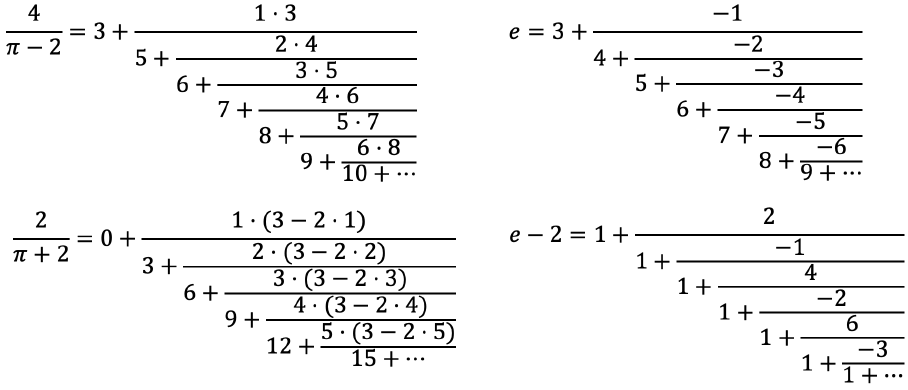Our MITM-RF algorithm was able to produce several novel conjectures, for example:
Conjectures 1-4:
Sample of automatically generated conjectures for mathematical formulas of funda-
mental constants, as generated by our proposed Ramanujan Machine by applying the MITM-RF algorithm.
All these results are previously unknown conjectures to the best of our knowledge. Both results for
π
converge exponentially and both results for
e
converge super-exponentially. See Table 3 in the Appendix for
additional results from our algorithms along with their convergence rates, which we separate to previously
known formulas and new formulas.
One may wonder whether the conjectures discovered by this work are indeed mathematical identities or
merely mathematical coincidences that breakdown once enough digits are calculated. However, the method
employed in this work makes it fairly unlikely for the conjectures to breakdown. For an enumeration space
of 10
9
and result accuracy of more than 50 digits, the probability of ﬁnding a random match is smaller
than 10
40
. This minuscule probability makes us believe that the new conjectures are truths awaiting a
rigorous proof by the mathematical community. In the past, the development of such proofs led to new
discoveries, such as the consequences on number theory of the proof of Fermat’s last theorem [
9
]. We
believe and hope that proofs of these new conjectures will lead to new discoveries in the future.
After discovering dozens of GCFs we observed empirically that there is a relationship between the
ratio of the polynomial order of
a
n
and
b
n
and the rate at which the formula converges as a function of
the number of iterations. This relationship was also proven rigorously in the Appendix.
In contrast to the method we present, many known RFs of fundamental constants were discovered by
conventional mathematical proofs, i.e. sequential logical steps derived from known properties of these
constants. For example, several RFs of
e
and
π
were generated using the Taylor expansion of the exponent
and the trigonometric functions and using Euler’s continued fraction formula [
10
], which connects an
inﬁnite sum and an inﬁnite GCF. In our work, we aim to reverse this process, ﬁnding new RFs for
the fundamental constants using their numerical data alone, without any prior knowledge about their
mathematical structure. Each RF may enable reverse-engineering of the mathematical structure that
produces the RF, and provide new insight on the ﬁeld. Our approach is especially powerful in cases of
empirical constants, such as the Feigenbaum constant from chaos theory (Table 2), which are derived
numerically from simulations and have no analytic representation.
Given the success of our approach in ﬁnding new RFs, there are many additional avenues for more
advanced algorithms and future research. Inspired by worldwide collaborative eﬀorts in mathematics such as
the Great Internet Mersenne Prime Search (GIMPS) we launch the initiative
www.RamanujanMachine.com
,
dedicated for ﬁnding new RFs for fundamental constants. The general community can donate computational
time to ﬁnd RFs, propose mathematical proofs for conjectured RFs, or suggest new algorithms for ﬁnding
3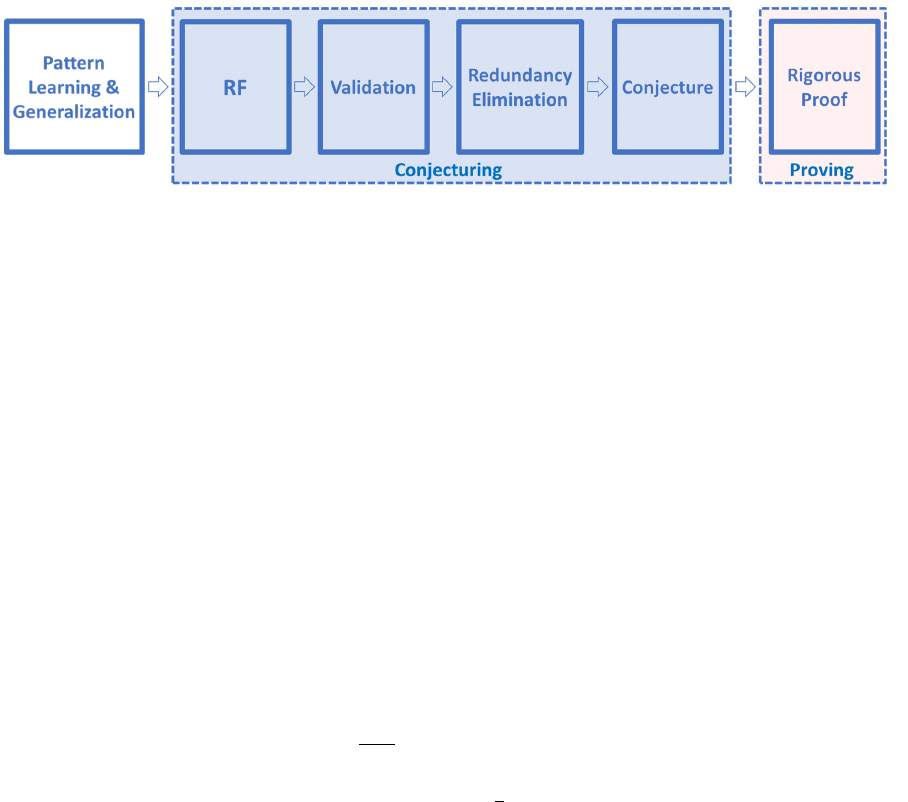them (see Appendix Section 4).
2 Related Work
Stated in an oversimpliﬁed manner, the process of mathematical research usually includes two main steps:
conjecturing and proving (as in Fig. 1). It is the latter step that was studied extensively in the computer
science literature and is known as Automated Theorem Proving (ATP), which focuses on proving
existing
conjectures
- fundamentally diﬀerent from our work that focuses on generating
new conjectures
. In
ATP, algorithms already proved many theorems [
11
], most notably the Four Color Theorem [
12
], the
Lorenz attractor problem [
13
], the Keppler Conjecture on the density of sphere packing [
14
], as well as
proving a conjectured identity for
ζ
(4) [
15
], and recent results [
16
,
17
]. In contrast, it is the "conjecturing"
step of the process of mathematical research that is the focus of this paper: automatic conjecturing of
RFs.
Figure 1:
Conceptual ﬂow of our Ramanujan Machine. First, through various approaches of pattern
learning & generalization (Section 5.1), we can generate a space of RF conjectures, e.g. GCFs. We
then apply a search algorithm, validate potential conjectures, and remove redundant results. Finally,
the validated results form mathematical conjectures that need to be proven analytically, thus closing a
complete research endeavour from pattern generation to a proof and potentially a new mathematical
insight.
Proposing conjectures is often times more signiﬁcant than proving them. For this reason some of the
most original mathematicians and scientists are known for their famous
unsolved conjectures
rather
than for their solutions to other problems, like Fermat’s last theorem, Hilbert’s problems, Landau’s
problems, Hardy-Littlewood prime tuple conjecture, Birch-Swinnerton-Dyer conjecture, and of course
the Riemann Hypothesis [
9
,
18
,
19
,
20
,
21
]. Maybe the most famous example is Ramanujan, who posed
dozens of conjectures involving fundamental constants and considered them to be revelations from one of
his goddesses [
22
]. In our work,
we aim to automate the process of conjectures generation
. We
demonstrate this concept by providing new conjectures for fundamental constants.
3 The Meet-In-The-Middle-RF Algorithm
Given a fundamental constant c (e.g. c = π), our goal is to learn a set of four polynomials (α,β,γ,δ):
γ(c)
δ(c)
= f
i
(GCF(α, β)) (3)
for
{f
i
}
a given set of functions (e.g.
f
1
(
x
) =
x, f
2
(
x
) =
1
x
, . . .
), where
GCF
(
α, β
) means the generalized
continued fraction with the partial numerator and denominator
a
n
=
α
(
n
) ;
b
n
=
β
(
n
) respectively as
deﬁned in Eq. (2). α, β, γ and δ are integer polynomials.
As showcased in Fig. 2, we start by enumerating over the two sides of Eq.
(3)
and successively
4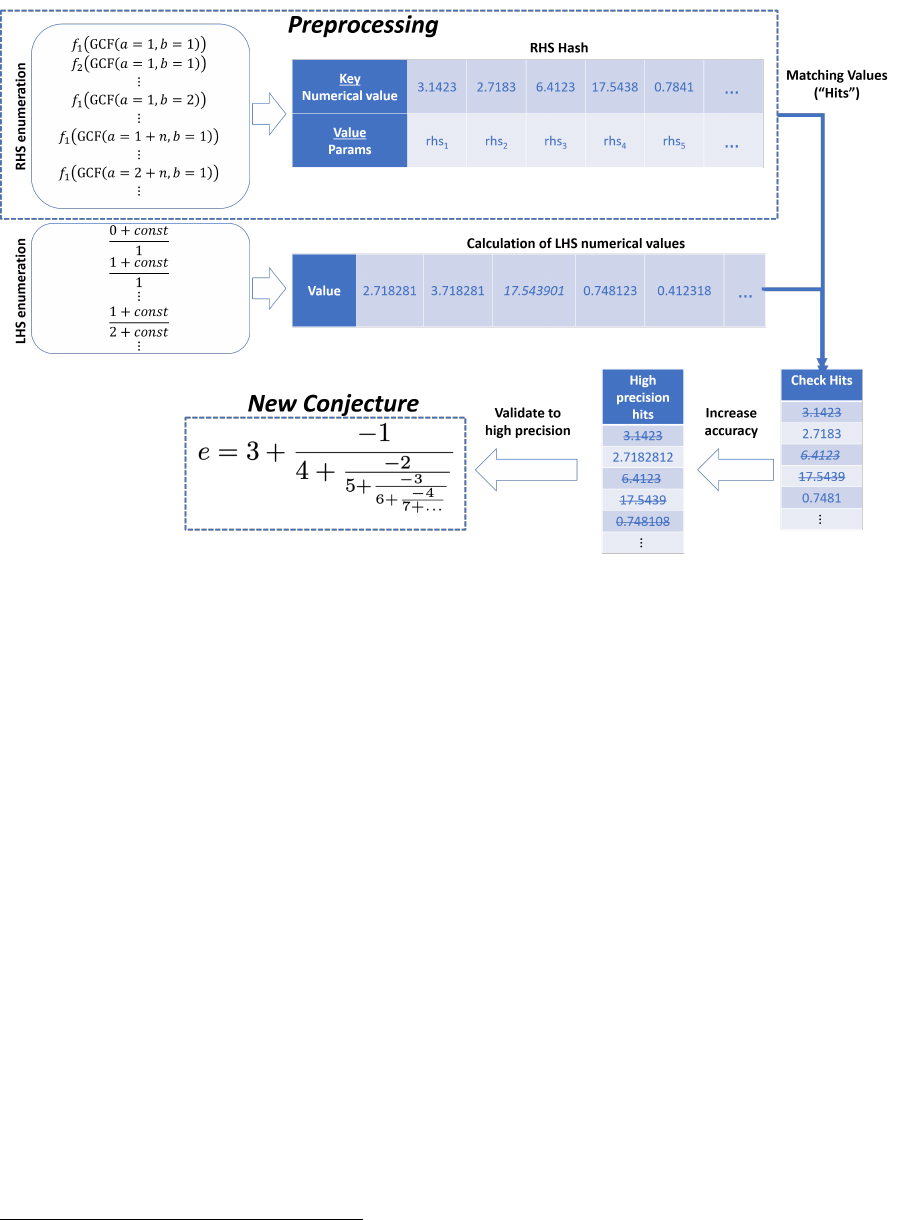Figure 2:
The Meet-In-The-Middle (MITM-RF) method: ﬁrst we enumerate RHS to a low precision,
values are stored in a hash-table. Then we enumerate LHS to a low precision and search for matches.
The matches are reevaluated to a higher precision and compared again. The process is repeated until a
speciﬁed, arbitrary decimal precision is reached, thus reducing false positives. The ﬁnal results are then
posed as new conjectures.
generating many diﬀerent integer polynomials for
α, β, γ, δ
2
. We calculate the Right-Hand-Side (RHS) of
each instance up to a limited number of iterations and store the results in a hash-table. We continue by
evaluating the Left-Hand-Side (LHS) up to a pre-selected decimal point. We attempt to then locate each
result from the LHS in the hash-table with the RHS results, where successful attempts are considered as
candidate solutions, and will be referred to as "hits".
Since the LHS and RHS calculations are performed up to a limited precision, several of the hits are
bound to be false positives. We then eliminate these false positives by calculating the rational function to
an arbitrary precision to reduce the likelihood that the equality is coincidental as shown in Fig. 3.
A naive enumeration method is very computationally intensive with time complexity of
O
(
MN
), where
M
and
N
are the LHS and RHS space size respectively, and space complexity of
O
(1). Since calculating
the RHS is more computationally costly, we store the RHS in the hash-table in order to signiﬁcantly
reduce computation time at the expense of space. This makes the algorithm’s time complexity
O
(
M
+
N
)
and its space complexity
O
(
N
). Moreover, the hash-table of the GCF (RHS) can be saved and reused for
further LHS enumerations, reducing future enumeration durations by a signiﬁcant amount.
We also generalize the aforementioned algorithm to allow for
α
and
β
to be interlaced sequences, i.e.
they may consist of multiple integer polynomials. The most simple example of a non-trivial interlaced
sequence is a sequence for which even values of
n
are equal to one polynomial and odd values of
n
are
equal to a diﬀerent polynomial. For results and details see Appendix Section 1 and the MITM-RF code
on www.RamanujanM achine.com.
2
We delete instances which produce trivial results like
γ
= 3
· δ
or
α
= 0 and instances whose
β
polynomial has zero roots,
which result in a ﬁnite GCF, necessarily representing a rational number.
5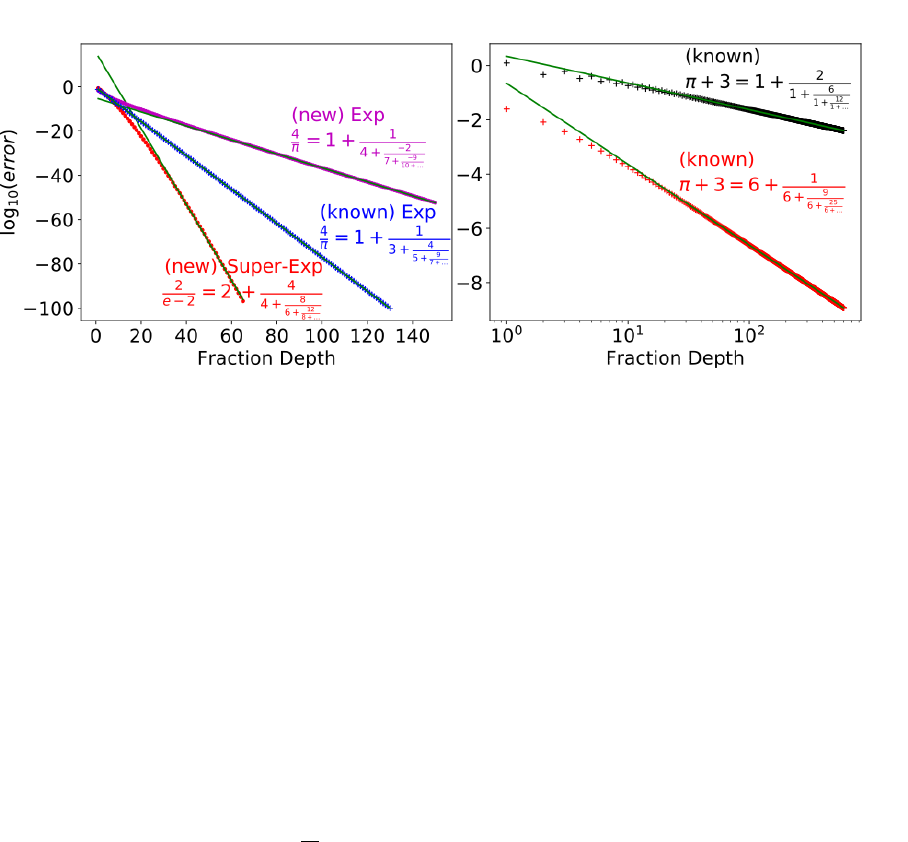Figure 3:
Convergence rate of the GCFs found by our method. Plots of the absolute diﬀerence between
the GCFs and the corresponding fundamental constant (i.e. the error) vs. the depth of the GCF. All the
results were found by our MITM-GCF algorithm. On the left are GCFs that converge exponentially/super-
exponentially (validated numerically), and on the right are GCFs which converge polynomially. The vast
majority of previously known results for
π
converge polynomially, while all of our newly found results
converge exponentially or super-exponentially.
With our proposed MITM algorithm we were able to discover new regular GCFs for fundamental
constants other than those previously known. However, seeing how successful our algorithm was despite
being relatively simplistic, we believe there is still ample room for new results, which should follow by
leveraging more sophisticated algorithms and more precise techniques, thus discovering hidden truths
about fundamental constants that may be considered to be more exotic than
π
and
e
perhaps with
formulas that are more complex than the GCFs that were used in this work.
3.1 Other Fundamental Constants with the MITM-RF
We also studied other fundamental constants of more exotic nature than
π
and
e
and found two new GCFs
for Apéry’s constant
ζ
(3) =
P
n=1
1
n
3
. Note that the MITM-RF algorithm does not need to use any prior
knowledge on the fundamental constant. However, there is a vast body of research on the properties of
many fundamental constants from which various structures can be inferred. Hence when aiming for such a
constant, one promising way to utilize such prior knowledge is to study other formulas of the fundamental
constant, in attempt to ﬁnd a common element and use that as a prior for the MITM-RF algorithm.
Such an approach can reduce dramatically the enumeration space and the computational complexity, thus
improving the chance for ﬁnding possible solutions. As a proof of concept of this approach for the Apéry
constant, consider formulas 1 & 2.
6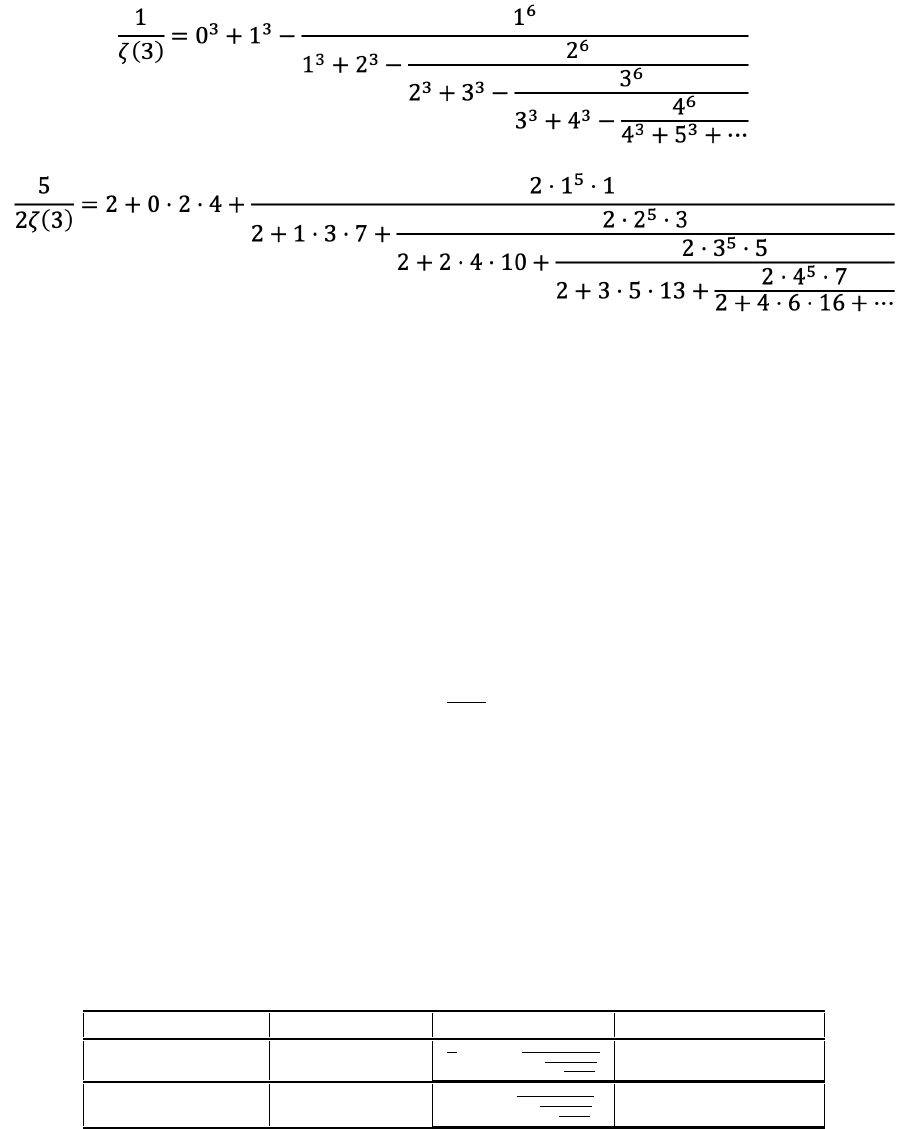Formulas 1 & 2
: New formulas for
ζ
(3) discovered using prior knowledge incorporated into MITM. The
top formula converges polynomially while to bottom converges exponentially. See Appendix Section 2 and
the attached code for details.
4 Descent&Repel
We propose a GD optimization method and demonstrate its success in ﬁnding RFs, and compare it with
the MITM-RF method. The MITM-RF method, although proved successful, is not trivially scalable. This
issue can be targeted by either a more sophisticated variant or by switching to an optimization based
method, as is done by the following algorithm.
As explained in Section 3, we want to ﬁnd integral solutions to Eq.
(3)
. This can also be written as
the following constrained optimization problem:
minimize
α, β, γ, δ
L =
γ(π)
δ(π)
GCF(α, β)
.
subject to {α, β, γ, δ} Z[x]
(4)
Solving this optimization problem with GD appears implausible, since we are only satisﬁed with global
minima without any error and the solutions must be integers. However, we found an important feature
of the loss landscape of the described problem that allows it to be solved with a slightly modiﬁed GD
that we name ’Descent&Repel’ (Fig. 4, example of results in Table 1). The minima are not 0-dimensional
points but (
d
1)-dimensional manifolds with
d
being the number of optimization variables as would be
expected given the single constraint. Moreover, we observed empirically that all minima are global and
their errors are zero, therefore any GD process will result in a solution with
L
= 0. It is well known that
any real number can be expressed as a simple continued fraction [
23
], and the aforementioned feature
hints that this may also be true for GCFs with integer polynomials.
Convergence Known / New Formula Polynomials
Exponential known
4
π
= 1 +
1
3+
4
5+
9
7+...
a
n
= 1 + 2n, b
n
= n
2
Super-Exponential new e = 3 +
1
4+
2
5+
3
6+...
a
n
= 3 + n, b
n
= n
Table 1: RFs for π and e found in a proof-of-concept run of the Descent&Repel algorithm.
We chose the variables of the optimization problem as the coeﬃcients of the
α, β, γ, δ
polynomials in
Eq.
(4)
. The optimization problem is initiated with a large set of points, speciﬁcally in the examples we
7